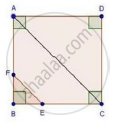Share

Books Shortlist
Your shortlist is empty

# Solution for Abcd is a Square. F is the Mid-point of Ab. Be is One Third of Bc. If the Area of δFbe = 108 Cm2, Find the Length of Ac. - CBSE Class 10 - Mathematics

ConceptApplication of Pythagoras Theorem in Acute Angle and Obtuse Angle

#### Question

ABCD is a square. F is the mid-point of AB. BE is one third of BC. If the area of ΔFBE = 108 cm2, find the length of AC.

#### SolutionSince, ABCD is a square

Then, AB = BC = CD = DA = x cm

Since, F is the mid-point of AB

Then, AF = FB = x/2cm

Since, BE is one third of BC

Then, BE = x/3cm

We have, area of ΔFBE = 108 cm2

rArr1/2xxBExxFB=108

rArr1/2xx x/2xx x/3=108

⇒ 𝑥2 = 108 × 2 × 3 × 2

⇒ 𝑥2 = 1296

rArrx=sqrt1296=36cm

In ΔABC, by pythagoras theorem AC2 = AB2 + BC2

⇒ AC2 = 𝑥2 + 𝑥2

⇒ AC2 = 2𝑥2

⇒ AC2 = 2 × (36)2

⇒ AC = 36sqrt2 = 36 × 1.414 = 50.904 cm

Is there an error in this question or solution?

#### Video TutorialsVIEW ALL 

Solution for question: Abcd is a Square. F is the Mid-point of Ab. Be is One Third of Bc. If the Area of δFbe = 108 Cm2, Find the Length of Ac. concept: Application of Pythagoras Theorem in Acute Angle and Obtuse Angle. For the course CBSE
S